# Edge-decompositions of graphs with high minimum degree

When can the edge set of a graph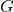be partitioned into triangles? Two obvious necessary conditions are that the total number of edges is divisible by 3 and the degree of every vertex is even. We call these conditions triangle divisibility. Triangle divisibility is not a sufficient condition for triangle decomposition (consider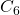), but it is sufficient ifis complete. So we would like to know how far from completecan be and triangle divisibility still remain sufficient for triangle decomposition. Nash-Williams conjectured that minimum degree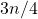(where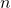is the number of vertices of) should suffice for large. In this paper we prove that every triangle divisible graph with minimum degree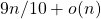has a triangle decomposition. We also prove similar results with any graphin place of triangles.

The proof uses the absorbing method. It is very easy to remove triangles at the beginning of the process, but very hard at the end. So we make use of the flexibility we have at the beginning to make a plan for dealing with a small remainder. The key idea is that given a possible remainder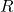we can find a graph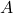such thatand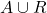both have triangle decompositions. By reserving sufficiently many such A at the start of the process we know that we will be able to solve our problems at the end.